### Splines and Spline Bases

This section provides details about the construction of spline bases with the EFFECT statement. A spline function is a piecewise polynomial function in which the individual polynomials have the same degree and connect smoothly at join points whose abscissa values, referred to as knots, are prespecified. You can use spline functions to fit curves to a wide variety of data.

A spline of degree 0 is a step function with steps located at the knots. A spline of degree 1 is a piecewise linear function where the lines connect at the knots. A spline of degree 2 is a piecewise quadratic curve whose values and slopes coincide at the knots. A spline of degree 3 is a piecewise cubic curve whose values, slopes, and curvature coincide at the knots. Visually, a cubic spline is a smooth curve, and it is the most commonly used spline when a smooth fit is desired. Note that when no knots are used, splines of degree d are simply polynomials of degree d.

More formally, suppose you specify knots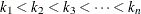. Then a spline of degree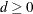is a function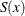with d – 1 continuous derivatives such that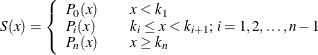where each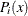is a polynomial of degree d. The requirement thathas d – 1continuous derivatives is satisfied by requiring that the function values and all derivatives up to order d – 1 of the adjacent polynomials at each knot match.

A counting argument yields the number of parameters that define a spline with n knots. There are n + 1 polynomials of degree d, giving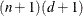coefficients. However, there are d restrictions at each of the n knots, so the number of free parameters is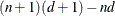= n + d + 1. In mathematical terminology this says that the dimension of the vector space of splines of degree d on n distinct knots is n + d + 1. If you have n + d + 1 basis vectors, then you can fit a curve to your data by regressing your dependent variable by using this basis for the corresponding design matrix columns. In this context, such a spline is known as a regression spline. The EFFECT statement provides a simple mechanism for obtaining such a basis.

If you remove the restriction that the knots of a spline must be distinct and allow repeated knots, then you can obtain functions with less smoothness and even discontinuities at the repeated knot location. For a spline of degree d and a repeated knot with multiplicity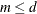, the piecewise polynomials that join such a knot are required to have only dm matching derivatives. Note that this increases the number of free parameters by m – 1 but also decreases the number of distinct knots by m – 1. Hence the dimension of the vector space of splines of degree d with n knots is still n + d + 1, provided that any repeated knot has a multiplicity less than or equal to d.

The EFFECT statement provides support for the commonly used truncated power function basis and B-spline basis. With exact arithmetic and by using the complete basis, you obtain the same fit with either of these bases. The following sections provide details about constructing spline bases for the space of splines of degree d with n knots that satisfies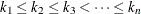.